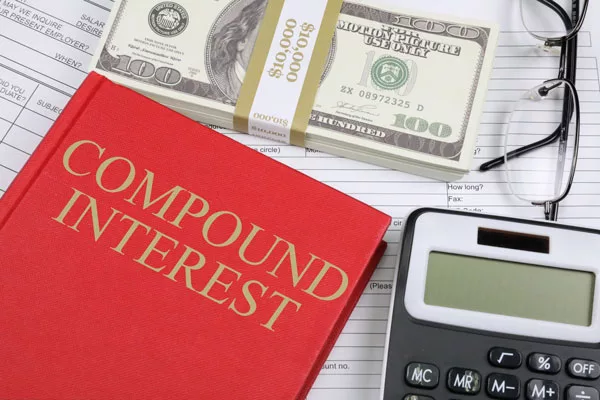# Compound Interest Calculator

Discover the transformative power of compound interest on your savings and investment balances.

Future Value: $0.00 ## Understanding Compound Interest The reason to use a compound interest calculator: Compound interest is a simple concept for savers: It represents the added interest on your initial amount as well as the accumulated interest over time. This phenomenon accelerates the growth of your savings. When you have an account that benefits from compound interest, like a regular savings account, the earned interest is combined with the main amount after each compounding interval, which could be daily or monthly. Every time this happens, the total amount in the account increases. Using the principles of compound interest, the subsequent compounding periods generate even more interest. Consider this: If you deposit$10,000 in a savings account with an annual rate of 4%, compounded daily, the interest for the first year would be $408, the next year$425, and it continues to increase. By the end of a decade, the accumulated interest would be approximately $4,918. However, it’s essential to note that this is a hypothetical scenario. For long-term financial goals, there are more advantageous options than just savings accounts, such as Roth or traditional IRAs and Certificates of Deposit (CDs). ## The Power of Compounded Returns in Investments Investing in the stock market doesn’t guarantee a fixed interest rate. Instead, your returns are determined by the fluctuating value of your investments. An increase in your investment’s value means you’ve earned a profit. By reinvesting your earnings in the market, the returns on those earnings also start to compound, mirroring the effects of compound interest. Imagine investing$10,000 in a mutual fund that yields a 6% return in a year. This would mean a profit of $600, making your total$10,600. If the next year also sees a 6% average return, your investment’s value would rise to $11,236. Over extended periods, the growth can be substantial. For instance, maintaining that$10,000 in a retirement fund for 30 years with an average 6% return annually would balloon the amount to over $57,000. It’s crucial to remember that investment returns can be unpredictable, varying from year to year or even day by day. Short-term investments, especially riskier ones like stocks, might sometimes decline in value. However, historically, a well-balanced growth portfolio has demonstrated an average annual return of about 6%. The magic of compounding can significantly boost your long-term financial aspirations, especially if you’re patient and let it work its wonders over extended periods. The potential earnings can far exceed your initial investment. ## Questions & Answers Q: What is compound interest? A: Compound interest is the interest earned on both the initial principal amount and the accumulated interest from previous periods. It allows savings or investments to grow at a faster rate compared to simple interest, which is calculated only on the principal amount. Q: How does compound interest work? A: Compound interest works by adding the interest earned in a specific period to the principal amount. This new total then becomes the basis for calculating interest in the next period. This process repeats, leading to an exponential growth of the invested amount over time. Q: How do you calculate compound interest? A: Compound interest can be calculated using the formula: A = P(1 + rn)nt Where: • A is the future value of the investment/loan, including interest. • P is the principal investment/loan amount. • r is the annual interest rate (as a decimal). • n is the number of times interest is compounded per year. • t is the number of years the money is invested/borrowed for. Q: What is the compound interest formula, with an example? A: The compound interest formula is: A = P(1 + rn)nt For example, if you deposit$5,000 in a savings account that earns a 3% annual interest rate and compounds monthly, you’d calculate

A = $5,000(1 + \frac{0.03}{12})^{12 \times 1} The ending balance after a year would be approximately$5,152.

Q: Why is compound interest referred to as the “eighth wonder of the world”?

A: Compound interest is often called the “eighth wonder of the world” because of its ability to exponentially grow savings or investments over time. The power of compounding allows even small investments to grow significantly when given enough time.

Q: How often is interest typically compounded?

A: Interest can be compounded on various schedules, including daily, monthly, quarterly, or annually. The frequency of compounding can significantly impact the total amount of interest earned.

Q: How does the frequency of compounding affect the amount of compound interest?

A: The more frequently interest is compounded, the greater the amount of compound interest will be over time. For instance, interest that is compounded daily will yield a higher amount than interest compounded annually, assuming the same interest rate and principal.

Q: What’s the difference between simple and compound interest?

A: Simple interest is calculated only on the principal amount, or on the portion of the principal amount which remains unpaid. Compound interest, on the other hand, is calculated on the initial principal, which also includes all the accumulated interest from previous periods on a deposit or loan.

Q: Can compound interest work against me?

A: Yes, while compound interest can significantly grow savings when you’re the investor, it can also accumulate rapidly on debts like credit card balances or loans. When you owe money, compound interest can result in you paying much more over time if you don’t manage and reduce your debts.

Q: Are there any online tools to help me calculate compound interest?

A: Yes, there are numerous online compound interest calculators that allow you to input your principal amount, interest rate, compounding frequency, and duration to get an estimate of your future balance.